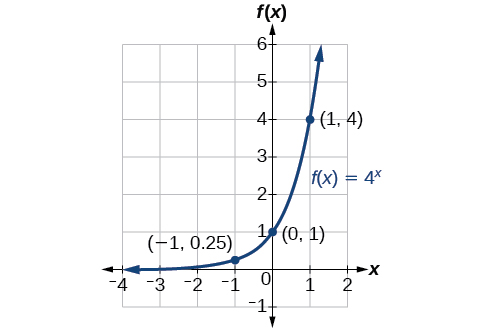# 6.2 Graphs of exponential functions  (Page 2/6)

 Page 2 / 6

## Sketching the graph of an exponential function of the form f ( x ) = b x

Sketch a graph of $\text{\hspace{0.17em}}f\left(x\right)={0.25}^{x}.\text{\hspace{0.17em}}$ State the domain, range, and asymptote.

Before graphing, identify the behavior and create a table of points for the graph.

• Since $\text{\hspace{0.17em}}b=0.25\text{\hspace{0.17em}}$ is between zero and one, we know the function is decreasing. The left tail of the graph will increase without bound, and the right tail will approach the asymptote $\text{\hspace{0.17em}}y=0.$
• Create a table of points as in [link] .  $x$ $-3$ $-2$ $-1$ $0$ $1$ $2$ $3$ $f\left(x\right)={0.25}^{x}$ $64$ $16$ $4$ $1$ $0.25$ $0.0625$ $0.015625$
• Plot the y -intercept, $\text{\hspace{0.17em}}\left(0,1\right),$ along with two other points. We can use $\text{\hspace{0.17em}}\left(-1,4\right)\text{\hspace{0.17em}}$ and $\text{\hspace{0.17em}}\left(1,0.25\right).$

Draw a smooth curve connecting the points as in [link] .

The domain is $\text{\hspace{0.17em}}\left(-\infty ,\infty \right);\text{\hspace{0.17em}}$ the range is $\text{\hspace{0.17em}}\left(0,\infty \right);\text{\hspace{0.17em}}$ the horizontal asymptote is $\text{\hspace{0.17em}}y=0.$

Sketch the graph of $\text{\hspace{0.17em}}f\left(x\right)={4}^{x}.\text{\hspace{0.17em}}$ State the domain, range, and asymptote.

The domain is $\text{\hspace{0.17em}}\left(-\infty ,\infty \right);\text{\hspace{0.17em}}$ the range is $\text{\hspace{0.17em}}\left(0,\infty \right);\text{\hspace{0.17em}}$ the horizontal asymptote is $\text{\hspace{0.17em}}y=0.$## Graphing transformations of exponential functions

Transformations of exponential graphs behave similarly to those of other functions. Just as with other parent functions, we can apply the four types of transformations—shifts, reflections, stretches, and compressions—to the parent function $\text{\hspace{0.17em}}f\left(x\right)={b}^{x}\text{\hspace{0.17em}}$ without loss of shape. For instance, just as the quadratic function maintains its parabolic shape when shifted, reflected, stretched, or compressed, the exponential function also maintains its general shape regardless of the transformations applied.

## Graphing a vertical shift

The first transformation occurs when we add a constant $\text{\hspace{0.17em}}d\text{\hspace{0.17em}}$ to the parent function $\text{\hspace{0.17em}}f\left(x\right)={b}^{x},$ giving us a vertical shift     $\text{\hspace{0.17em}}d\text{\hspace{0.17em}}$ units in the same direction as the sign. For example, if we begin by graphing a parent function, $\text{\hspace{0.17em}}f\left(x\right)={2}^{x},$ we can then graph two vertical shifts alongside it, using $\text{\hspace{0.17em}}d=3:\text{\hspace{0.17em}}$ the upward shift, $\text{\hspace{0.17em}}g\left(x\right)={2}^{x}+3\text{\hspace{0.17em}}$ and the downward shift, $\text{\hspace{0.17em}}h\left(x\right)={2}^{x}-3.\text{\hspace{0.17em}}$ Both vertical shifts are shown in [link] .

Observe the results of shifting $\text{\hspace{0.17em}}f\left(x\right)={2}^{x}\text{\hspace{0.17em}}$ vertically:

• The domain, $\text{\hspace{0.17em}}\left(-\infty ,\infty \right)\text{\hspace{0.17em}}$ remains unchanged.
• When the function is shifted up $\text{\hspace{0.17em}}3\text{\hspace{0.17em}}$ units to $\text{\hspace{0.17em}}g\left(x\right)={2}^{x}+3:$
• The y- intercept shifts up $\text{\hspace{0.17em}}3\text{\hspace{0.17em}}$ units to $\text{\hspace{0.17em}}\left(0,4\right).$
• The asymptote shifts up $\text{\hspace{0.17em}}3\text{\hspace{0.17em}}$ units to $\text{\hspace{0.17em}}y=3.$
• The range becomes $\text{\hspace{0.17em}}\left(3,\infty \right).$
• When the function is shifted down $\text{\hspace{0.17em}}3\text{\hspace{0.17em}}$ units to $\text{\hspace{0.17em}}h\left(x\right)={2}^{x}-3:$
• The y- intercept shifts down $\text{\hspace{0.17em}}3\text{\hspace{0.17em}}$ units to $\text{\hspace{0.17em}}\left(0,-2\right).$
• The asymptote also shifts down $\text{\hspace{0.17em}}3\text{\hspace{0.17em}}$ units to $\text{\hspace{0.17em}}y=-3.$
• The range becomes $\text{\hspace{0.17em}}\left(-3,\infty \right).$

## Graphing a horizontal shift

The next transformation occurs when we add a constant $\text{\hspace{0.17em}}c\text{\hspace{0.17em}}$ to the input of the parent function $\text{\hspace{0.17em}}f\left(x\right)={b}^{x},$ giving us a horizontal shift     $\text{\hspace{0.17em}}c\text{\hspace{0.17em}}$ units in the opposite direction of the sign. For example, if we begin by graphing the parent function $\text{\hspace{0.17em}}f\left(x\right)={2}^{x},$ we can then graph two horizontal shifts alongside it, using $\text{\hspace{0.17em}}c=3:\text{\hspace{0.17em}}$ the shift left, $\text{\hspace{0.17em}}g\left(x\right)={2}^{x+3},$ and the shift right, $\text{\hspace{0.17em}}h\left(x\right)={2}^{x-3}.\text{\hspace{0.17em}}$ Both horizontal shifts are shown in [link] .

Observe the results of shifting $\text{\hspace{0.17em}}f\left(x\right)={2}^{x}\text{\hspace{0.17em}}$ horizontally:

• The domain, $\text{\hspace{0.17em}}\left(-\infty ,\infty \right),$ remains unchanged.
• The asymptote, $\text{\hspace{0.17em}}y=0,$ remains unchanged.
• The y- intercept shifts such that:
• When the function is shifted left $\text{\hspace{0.17em}}3\text{\hspace{0.17em}}$ units to $\text{\hspace{0.17em}}g\left(x\right)={2}^{x+3},$ the y -intercept becomes $\text{\hspace{0.17em}}\left(0,8\right).\text{\hspace{0.17em}}$ This is because $\text{\hspace{0.17em}}{2}^{x+3}=\left(8\right){2}^{x},$ so the initial value of the function is $\text{\hspace{0.17em}}8.$
• When the function is shifted right $\text{\hspace{0.17em}}3\text{\hspace{0.17em}}$ units to $\text{\hspace{0.17em}}h\left(x\right)={2}^{x-3},$ the y -intercept becomes $\text{\hspace{0.17em}}\left(0,\frac{1}{8}\right).\text{\hspace{0.17em}}$ Again, see that $\text{\hspace{0.17em}}{2}^{x-3}=\left(\frac{1}{8}\right){2}^{x},$ so the initial value of the function is $\text{\hspace{0.17em}}\frac{1}{8}.$

root under 3-root under 2 by 5 y square
The sum of the first n terms of a certain series is 2^n-1, Show that , this series is Geometric and Find the formula of the n^th
cosA\1+sinA=secA-tanA
why two x + seven is equal to nineteen.
The numbers cannot be combined with the x
Othman
2x + 7 =19
humberto
2x +7=19. 2x=19 - 7 2x=12 x=6
Yvonne
because x is 6
SAIDI
what is the best practice that will address the issue on this topic? anyone who can help me. i'm working on my action research.
simplify each radical by removing as many factors as possible (a) √75
how is infinity bidder from undefined?
what is the value of x in 4x-2+3
give the complete question
Shanky
4x=3-2 4x=1 x=1+4 x=5 5x
Olaiya
hi can you give another equation I'd like to solve it
Daniel
what is the value of x in 4x-2+3
Olaiya
if 4x-2+3 = 0 then 4x = 2-3 4x = -1 x = -(1÷4) is the answer.
Jacob
4x-2+3 4x=-3+2 4×=-1 4×/4=-1/4
LUTHO
then x=-1/4
LUTHO
4x-2+3 4x=-3+2 4x=-1 4x÷4=-1÷4 x=-1÷4
LUTHO
A research student is working with a culture of bacteria that doubles in size every twenty minutes. The initial population count was  1350  bacteria. Rounding to five significant digits, write an exponential equation representing this situation. To the nearest whole number, what is the population size after  3  hours?
v=lbh calculate the volume if i.l=5cm, b=2cm ,h=3cm
Need help with math
Peya
can you help me on this topic of Geometry if l help you
litshani
( cosec Q _ cot Q ) whole spuare = 1_cosQ / 1+cosQ
A guy wire for a suspension bridge runs from the ground diagonally to the top of the closest pylon to make a triangle. We can use the Pythagorean Theorem to find the length of guy wire needed. The square of the distance between the wire on the ground and the pylon on the ground is 90,000 feet. The square of the height of the pylon is 160,000 feet. So, the length of the guy wire can be found by evaluating √(90000+160000). What is the length of the guy wire?
the indicated sum of a sequence is known as
how do I attempted a trig number as a starter
cos 18 ____ sin 72 evaluateByByBy OpenStaxBy Anh DaoBy OpenStaxBy OpenStaxBy Jill ZerressenBy Brooke DelaneyByBy OpenStaxBy OpenStaxBy Stephen Voron